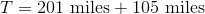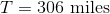# High School Math : How to do word problems where two quantities are unknown

## Example Questions

### Example Question #1 : How To Do Word Problems Where Two Quantities Are Unknown

A farmer hasunits of fence. If he uses this to build a square fence, what will be the area enclosed by the fence?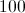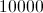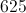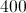Explanation:

Since we are looking at a square, the formula for the area of a square is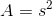.

Therefore we need to know the length of each side.

Since this is a square fence, then each of the four sides will be equal.

The amount of fence that the farmer has in the problem will become the perimeter of our square.

Since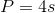when working with a square, for this problem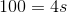.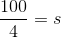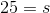Plug that into our original equation.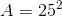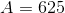### Example Question #2 : How To Do Word Problems Where Two Quantities Are Unknown

Rosie, Eileen and Sasha order a pizza. It is divided intoslices. Eileen eatsslice. Roseanne eats twice as many slices as Sasha.

If there areslices of pizza remaining, how many slices did Sasha eat?slicesslicesslicesslicessliceslices

Explanation:

Since the pizza began asslices and there are nowslices remaining, a total ofslices were eaten (because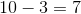). Of theeaten pieces, it is stated thatslice was eaten by Eileen. This means a total ofslices were eaten by Roseanne and Sasha combined. To find exactly how many slices Sasha ate, use the fact that Roseanne ate twice as many slices as Sasha. Write and solve forin the following equation, whereis the number of slices Sasha ate. Note that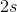stands for the number of slices Roseanne ate, since she ate twice as many slices as Sasha.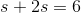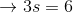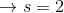slices

### Example Question #3 : How To Do Word Problems Where Two Quantities Are Unknown

A helicopter flies against the wind from city A to city B in 5 hours. The same helicopter returns from city B to city A, in the same direction as the wind, in 4 hours. Find the ratio of the speed of the helicopter to the speed of the wind.

7

10

8

11

9

9

Explanation:

First, let= speed of the helicopter,= speed of the wind, and= distance between city A and city B. Next, find the ratio ofto. Traveling against the wind: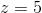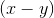. Traveling with the wind: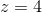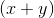. Finally, set the equations equal to one another: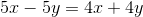.

Simplify the equation: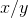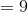.

### Example Question #4 : How To Do Word Problems Where Two Quantities Are Unknown

Michael hasred shirts andblue shirts, such that the ratio isred shirts for everyblue shirts. What is the minimum number of shirts he can have?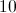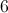There is insufficient information to answer the question.Explanation:

It really doesn't matter whatandare. What matters is the ratio given to us,red:blue. Let's assume that he can only have whole shirts. That means that the minimum number of red shirts he can have is, and the minimum number of blue is, giving us a total of.

### Example Question #5 : How To Do Word Problems Where Two Quantities Are Unknown

Two cars leave a city at the same time. One heads east at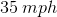and the other heads west at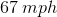. How far apart are they afterhours?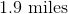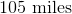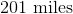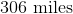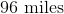Explanation:

Remember,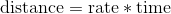. If we look at the car going east, this would mean: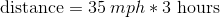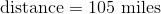If we look at the car going west, then: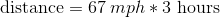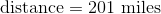Therefore, we need to add the two distances to find the total distance between them.